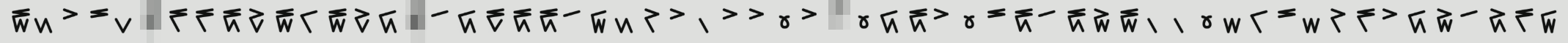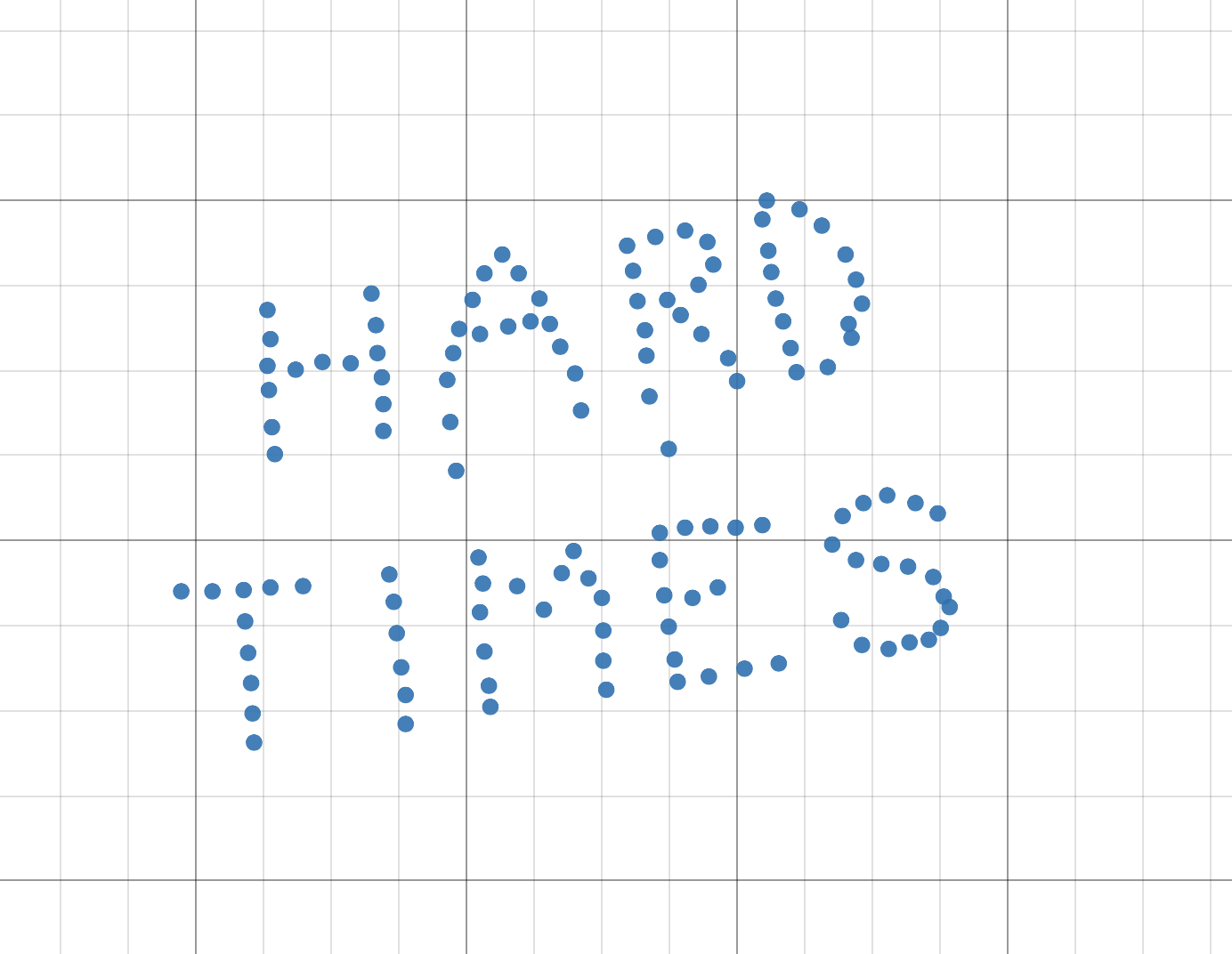# HackTM

Challenge Category Points
Count on me Crypto 467
OLD Times OSINT 424

# Crypto

#### 197 Points

I captured this encrypted message a while ago. Today, I got into their network and managed to take a snapshot of their key server. I don’t think more than 10k messages have been sent between when I captured the message and when I took the snapshot.

You can access the snapshot here: nc 138.68.67.161 60005

I solved this challenge with Hyperreality and the help of a stack exchange post. We are given access to a server which gives public, private key pairs. We have a ciphertext c. To solve this challenge, we generate new key pairs and use an algorithm to factor N using (e,d,n) and try to find a weakness in the implementation.

To factor N, I used the algorithm described by fgrieu here which I repeat

1. Compute f <- ed - 1 and express f = 2**s * t
2. Set i = s and a = 2
3. Compute b = a**t mod n
• If b == 1: set a to the next prime and jump to 3.
4. If i != 1:
• Compute c = b**2 mod N
• If c != 1 set b = c and i -= 1
• Jump to 4.
5. If b == N - 1: set a to next prime and jump to 3.
6. Computer p = gcd(N, b - 1) and q = N // q

Putting this into python, we can write an algorithm to factor generated keys. Note I discard any key pairs that have d < 0. Potentially there’s a way to use these, but as I don’t have \phi(N) I couldn’t see a quick way and it was easier to just grab a different key pair.

from sympy import nextprime
import math

def find_prime_factors(n,e,d):
k = e * d - 1
s = 0
t = k

while t % 2 == 0:
t = t // 2
s += 1

i = s
a = 2

while True:
b = pow(a,t,n)

if b == 1:
a = nextprime(a)
continue

while i != 1:
c = pow(b,2,n)
if c == 1:
break
else:
b = c
i -= 1

if b == n - 1:
a = nextprime(a)
continue

p = math.gcd(b-1, n)
q = n // p
return p, q

keys = [
((65537, 16730003700605898732103808147265049097309227731116112091966825592580794522488597118108918276114596284933863997925167175825551948545026542789031655603762767594028984696331306133063729472164129741174402731943970446813396896291870363659061827254054595300539660296594191052826127087049878251823692914198236061007), (4549013014706152484948805425702476080901634773768849924147410349265144245094325352773256689814335502044973929887338130723275946764001602033973848404093142468913140303660855127247147909135645926989846389684877266437028310259235212527602515443688484814503867603041563521965407000243692658755982940385673478273, 16730003700605898732103808147265049097309227731116112091966825592580794522488597118108918276114596284933863997925167175825551948545026542789031655603762767594028984696331306133063729472164129741174402731943970446813396896291870363659061827254054595300539660296594191052826127087049878251823692914198236061007)),
((65537, 144517755992564048861504174877857487852987341166328935206455623191881428189718009215250567859676443497706126512409112506483468354898393992286046087915659080819539156733582684190969034177936751150611020408774463197135796355334158803766859705325385107989926116428050293832482242072477621375482173139770930869421), (31054878277053870334567699083035033666991481539365707836985436339949435482182564410613451137034398183899100960896249926435550678884204076984976228025637830486164122358535037197188466113194006283698671271205473089863266402202388548638617988140763543550548546126891968521417488321501698697994048418712496082773, 144517755992564048861504174877857487852987341166328935206455623191881428189718009215250567859676443497706126512409112506483468354898393992286046087915659080819539156733582684190969034177936751150611020408774463197135796355334158803766859705325385107989926116428050293832482242072477621375482173139770930869421)),
((65537, 123741962902154205189909884965124653318102255507428661008324023486380505709197314715589325195416080106121685443970029442140815672039378844508982777903697232853456171326974565483936014318756930718266360727767369367994474730211277431252795244877611270845326337495473012176653152632601881293713941472626554833217), (15541146171592097027910161636143568083087411158302108866129286316376977012869113591162484332262229814509171809654352691430200555358898443156589975814049037029826267580422408827286358483357720841255938886100043208316256177732763374420025617386627628801933221633401610870745630145991479549460332955788507442461, 123741962902154205189909884965124653318102255507428661008324023486380505709197314715589325195416080106121685443970029442140815672039378844508982777903697232853456171326974565483936014318756930718266360727767369367994474730211277431252795244877611270845326337495473012176653152632601881293713941472626554833217))]

e = 65537

for k in keys:
n = k
d = k
if d > 0:
p, q = find_prime_factors(n,e,d)
if p == 1 or q == 1:
print('[-] Algorithm broken')
else:
print("[+] Prime pair found")
print("p = ", p)
print("q = ", q)
print("")


Running this script we get the following prime pairs

[+] Prime pair found
p =  1380623332297453118984873537786777519839182349276676087241139668719694587529609212189422107766171700904217158758941493834627126539787388156700567832193247
q =  12117717634661447128647943483912040772241097914126380240028878917605920543320951000813217299678214801720664141663955381289172887935222185768875580129864081

[+] Prime pair found
p =  12117717634661447128647943483912040772241097914126380240028878917605920543320951000813217299678214801720664141663955381289172887935222185768875580129865231
q =  11926153121375457949010884635428120250029675016450611713057458832154147128348531323843554423542842489299014770027236460611202063816127702713202828814511491

[+] Prime pair found
p =  10211655910202382811964911729055262513625584135244524229115154619070582543942720064521969215139126418488027025507905871989461676818719689776178201641556303
q =  12117717634661447128647943483912040772241097914126380240028878917605920543320951000813217299678214801720664141663955381289172887935222185768875580129865839


and we see that for each prime pair, one prime seems to be very close in value. Guessing that the algorithm is generating p as p = nextprime(p) we can find the prime factors of N by starting with one of our found p and going backwards towards 0 until we find a factor.

from Crypto.Util.number import inverse

p0 = 12117717634661447128647943483912040772241097914126380240028878917605920543320951000813217299678214801720664141663955381289172887935222185768875580129864081

n = 2318553827267041599931064141028026591078453523755133761420994537426231546233197332557815088229590256567177621743082082713100922775483908922217521567861530205737139513575691852244362271068595653732088709994411183164926098663772268120044065766077197167667585331637038825079142327613226776540743407081106744519
e = 65537
c = 2255296633936604604490193777189642999170921517383872458719910324954614900683697288325565056935796303372973284169167013060432104141786712034296127844869460366430567132977266285093487512605926172985342614713659881511775812329365735530831957367531121557358020217773884517112603921006673150910870383826703797655

while True:
if n % p0 == 0:
p = p0
q = n // p
phi = (p-1)*(q-1)
d = inverse(e,phi)
m = pow(c,d,n)
print(bytes.fromhex(format(m,'x')).decode('utf-8'))
break
p0 -= 2


#### Flag

HackTM{SanTa_ple@s3_TakE_mE_0ff_yOur_l1st_4f2d20ec18}

## Count on me

### 467 Points

CORRECTION: AES 256 is used. Not AES 128. Hint! To decrypt the message, you need a hex-string of 64 characters in length. Hint! WARNING: This challenge has been updated at 02-02-2020 15:00 UTC to fix a critical mistake.

We are given the source for the challenge

from Crypto.Cipher import AES
# this is a demo of the encyption / decryption proceess.

a = 'flagflagflagflag'
key = '1111111111111111111111111111111111111111111111111111111111111111'.decode('hex')
iv = '42042042042042042042042042042042'.decode('hex')

#encrypt
aes = AES.new(key,AES.MODE_CBC, iv)
c = aes.encrypt(a).encode("hex")
print c

#decrypt
aes = AES.new(key,AES.MODE_CBC, iv)
print aes.decrypt(c.decode("hex"))


together with all the challenge details

AES 256 CBC
iv: 42042042042042042042042042042042
ciphertext: 059fd04bca4152a5938262220f822ed6997f9b4d9334db02ea1223c231d4c73bfbac61e7f4bf1c48001dca2fe3a75c975b0284486398c019259f4fee7dda8fec


The key for the AES decrption is a .pngand the task of this challenge is to interpret this string as a len64 hex string.

Looking at each glyph it seemed reasonable to see this as a Vigesimal system. The lower half counting between 0-4 and the upper lines counting [0,5,10,15]. This means each glyph can be a number between 0,19.

Going left to right we obtain an array on base10 integers

glyphs = [19, 3, 10, 15, 2, ?, 16, 16, 18, 12, 19, 6, 19, 12, 8, ?, 5, 8, 17, 18, 18, 5, 9, 3, 11, 10, 1, 10, 10, 0, 10, ?, 0, 8, 18, 10, 0, 15, 18, 5, 18, 14, 19, 1, 1, 0, 4, 6, 15, 4, 11, 16, 10, 8, 14, 5, 13, 16, 9]


Where I have replaced the blurred glyphs with ?. The question is, how do we take these numbers and get a len 64 string? To test, I swap all ? = 0. The first thing I tried was joining each element to obtain one long int in base10 and converting to a hex string:

int_10 = int(''.join([str(i) for i in glyphs]))
>>> 1912238077151019519101301131115151171262422020013152031511518194401653166721318114717
int_16 = hex(int_10)[2:]
>>> 'fbfd6a5a0c04ee47556e2a9a42a7eb5f2280c8c7830703c5d41bbce3e0508caa29d59d'
len(int_16)
>>> 70


Then I tried converting each glyph to base16 and then concat:

concat_int_16 = ''.join([hex(i)[2:] for i in glyphs]
len(concat_int_16)
>>> 69


Neither of these were appropriate. I got stuck here for a while and solved some other challenges. Coming back later I had the idea of concating each glyph from base20. One way to do this would be to go through glyphs and replace {10,19} -> {a,j}, but I found it easier to just grab an int_2_base function from an old challenge and solve

import string
digs = string.digits + string.ascii_letters

def int2base(x, base):
if x < 0:
sign = -1
elif x == 0:
return digs
else:
sign = 1

x *= sign
digits = []

while x:
digits.append(digs[int(x % base)])
x = int(x / base)

if sign < 0:
digits.append('-')

digits.reverse()

return ''.join(digits)

glyphs = [19, 3, 10, 15, 2, 0, 16, 16, 18, 12, 19, 6, 19, 12, 8, 0, 5, 8, 17, 18, 18, 5, 9, 3, 11, 10, 1, 10, 10, 0, 10, 0, 0, 8, 18, 10, 0, 15, 18, 5, 18, 14, 19, 1, 1, 0, 4, 6, 15, 4, 11, 16, 10, 8, 14, 5, 13, 16, 9]

int_20 = ''.join([int2base(i, 20) for i in glyphs])
int_10 = int(int_20, 20)
int_16 = hex(int_10)[2:]

print(int_20)
print(int_10)
print(int_16)
print(len(int_16))

>>> 'j3af20ggicj6jc8058hii593ba1aa0a008ia0fi5iej11046f4bga8e5dg9'
>>> 55273615734144947969560678724501073228899919180366431845779064168750747885529
>>> '7a33c20284ab07c18b0100b75594af73c47005d27a90b86496f3bbe27c6e1fd9'
>>> 64


Now we’re getting there!! All that’s left is to go through all 20**3 options from the three missing glyphs and decode the ciphertext

## Python Implementation

from Crypto.Cipher import AES
from Crypto.Util.number import long_to_bytes
import string
digs = string.digits + string.ascii_letters

def int2base(x, base):
if x < 0:
sign = -1
elif x == 0:
return digs
else:
sign = 1

x *= sign
digits = []

while x:
digits.append(digs[int(x % base)])
x = int(x / base)

if sign < 0:
digits.append('-')

digits.reverse()

return ''.join(digits)

ciphertext = bytes.fromhex('059fd04bca4152a5938262220f822ed6997f9b4d9334db02ea1223c231d4c73bfbac61e7f4bf1c48001dca2fe3a75c975b0284486398c019259f4fee7dda8fec')
iv = bytes.fromhex('42042042042042042042042042042042')

for i in range(0,20):
for j in range(0,20):
for k in range(0,20):
glyphs = [19, 3, 10, 15, 2, i, 16, 16, 18, 12, 19, 6, 19, 12, 8, j, 5, 8, 17, 18, 18, 5, 9, 3, 11, 10, 1, 10, 10, 0, 10, k, 0, 8, 18, 10, 0, 15, 18, 5, 18, 14, 19, 1, 1, 0, 4, 6, 15, 4, 11, 16, 10, 8, 14, 5, 13, 16, 9]
bigint_20 = ''.join([int2base(i, 20) for i in glyphs])
bigint_10 = int(bigint_20,20)
key = long_to_bytes(bigint_10)
aes = AES.new(key,AES.MODE_CBC, iv)
plaintext = aes.decrypt(ciphertext)
if b'Hack' in plaintext:
print(plaintext)


#### Flag

HackTM{can_1_h@ve_y0ur_numb3r_5yst3m_??}

# OSINT

## OLD Times

### 424 Points

There are rumors that a group of people would like to overthrow the communist party. Therefore, an investigation was initiated under the leadership of Vlaicu Petronel. Be part of this ultra secret investigation, help the militia discover all secret locations and you will be rewarded.

Looking at the given text, the main thing that stands out is the name. A quick google gives twitter.com/PetronelVlaicu

There’s a few tweets that stand out. A photo which is tagged with another user.

There’s only one person the account follows:

They have a link in their bio:

Another tweet tells us that they “love Temple OS”

But I wasnt seeing much to go forward. Using wayback machine, I can look of snapshots of the account and I find a deleted tweet with the string:

1XhgPI0jpK8TjSMmSQ0z5Ozcu7EIIWhlXYQECJ7hFa20

This looks hopefull! Base64 against this doesn’t produce anything readable, but this Link Identifier by a2x2 tells use that this b64 is associated to a Google Doc

One part of the doc stands out more than others:

The local activity is under control. People seek their daily routine and have no doubts about the party, except for one man: Iovescu Marian - who goes by the name of E4gl3OfFr3ed0m

Googling this leads to a GitHub user with a single repository

Now we can go through old commits and look at the deleted data. In a line edit we find the url http://138.68.67.161:55555/

Accessing this account gives a forbidden response though. Looking deeper, we find an old .php file:

# spread_locations.php
<?php

$myfile = fopen("locations.txt", "r") or die("Unable to open file!");$locs = fread($myfile,filesize("locations.txt")); fclose($myfile);
$locs = explode("\n",$locs);

$reg =$_GET["region"];
if($reg < 129 &&$reg >= 0){
echo "<b>[".$reg."]:</b> "; echo$locs[\$reg];
}
else{
echo "<b>Intruder!</b>";
}

?>


I wrote a quick python script to get all the locations data:

import requests

locations = []

for x in range(0,129):
loc = page.text.split(' ')
l = loc
locations.append(l)

for l in locations:
print(l)


This gives the data

22.5277957,47.3561089
22.5497683,47.184652
22.5277957,47.0276192
22.538782,46.8851427
22.5607546,46.6669469
22.5827273,46.508391
22.7365359,47.0051481
22.9342898,47.0500808
23.14303,47.0425947
23.2968386,47.4527737
23.3297976,47.2667225
23.3407839,47.1024545
23.3737429,46.9601776
23.3847292,46.8024828
23.3847292,46.6443244
23.879114,46.6970954
24.2636355,47.6825664
24.8459109,46.7648681
25.1864871,47.7343156
25.351282,46.8475858
24.1317996,47.5715023
24.0439089,47.4156159
23.945032,47.2443522
23.9010867,47.1024545
23.8571414,46.945179
24.3844851,47.5715023
24.5382937,47.4230496
24.615198,47.2741771
24.6921023,47.1398328
24.8019656,46.9826676
24.0988406,47.2145105
24.3075808,47.2592668
24.4723757,47.2890833
25.2304324,47.5863245
25.2633914,47.4081812
25.318323,47.2368934
25.3293093,47.0874959
25.4831179,47.4156159
25.3952273,47.7860133
25.6149539,47.8229089
25.7797488,47.756478
25.8236941,47.6233616
25.7138308,47.5047505
25.5819949,47.3263302
25.7358035,47.2145105
25.9335574,47.072533
25.9994753,46.9376782
26.2192019,47.9996428
26.1862429,47.8892548
26.2301882,47.7047509
26.2521609,47.5789139
26.2851199,47.4230496
26.3400515,47.2890833
26.4609011,47.9481577
26.625696,47.8524065
26.8014773,47.6825664
26.8783816,47.5344284
26.3949832,47.1323592
26.9223269,47.3933087
26.82345,47.2741771
26.4389285,46.9901622
26.6696414,47.0201299
26.8454226,47.1921182
21.8905886,45.7009791
22.1213015,45.7009791
22.3520144,45.7086515
22.5497683,45.723993
22.7914675,45.7316622
23.4286746,45.8006377
24.0878542,45.9001182
24.7909792,45.9383326
25.4281863,46.0451929
25.6149539,46.0756865
25.8017214,46.0833073
25.988489,46.0756865
26.1862429,46.090927
22.3630007,45.5242242
22.3849734,45.3391904
22.406946,45.161297
22.4179324,44.9828465
22.4289187,44.8116333
23.4616335,45.6395623
23.4836062,45.4549076
23.5165652,45.2541805
23.5495242,45.0915349
23.5495242,44.9206461
24.1208132,45.7469975
24.9008425,45.7776553
24.0988406,45.5780782
24.1317996,45.3469122
24.1647585,45.1458017
24.1757449,45.0216875
24.9997195,45.6626015
25.0107058,45.4703187
25.0107058,45.2928371
25.0326785,45.1225508
24.5712527,45.5934555
24.7030886,45.8082963
24.3734988,45.7316622
25.4281863,45.8848251
25.4611453,45.6779557
25.4941042,45.4934274
25.5380496,45.3005653
25.5600222,45.1690431
25.7907351,45.2000169
26.054407,45.246446
26.3070925,45.2773776
25.6698855,45.6626015
25.8566531,45.723993
27.4826296,46.1594571
27.3178347,46.2203012
27.1090945,46.2658901
26.9333132,46.2203012
26.7795046,46.1442356
26.7026003,45.9765207
26.8783816,45.8848251
27.0651492,45.8618775
27.2629031,45.8465739
27.4496707,45.7853172
27.526575,45.6702792
27.5705203,45.6088287
27.5046023,45.4857255
27.4167117,45.4163615
27.2738894,45.4009357
27.1200808,45.3623528
26.9223269,45.3855057
26.7685183,45.5319208
23.9230593,46.410005
25.4941042,46.5386279


These coordinates are all too close to be interesting on a map, but we can plot them all on a plane using DesmosWhich gives us the flag!

#### Flag

HackTM{HARDTIMES}